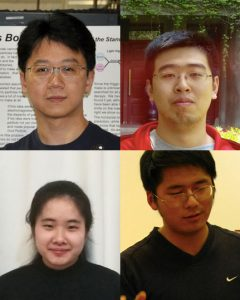## Light, heavy light and asymmetryComparison of the forward backward asymmetry in Z → μ+ μ for data (blue) and the best fit (red). The horizontal axis is the reconstructed mass of the muon pair; it is calculated from the energy and directions of the μ+ and μ.

One of the major steps forward in particle physics was the development by Feynman, Tomonaga, Schwinger, Dyson and others of a theory of quantized electromagnetic forces in the late 1940s. The result of quantization, the particle that carries the electromagnetic force, is the photon, and it is an example — the first example, historically — of the type of particle that we call a vector boson. We use the symbol γ (gamma) for this particle.

The weak nuclear force was discovered in the form of beta decay back in 1899 by Ernest Rutherford. Over the years, we came to realize that, like electromagnetic fields, these weak fields also have vector boson particles. Unlike electromagnetic forces, there are three different vector bosons for the weak force: One (the W+) has a positive charge, one (the W) has a negative charge, and the third one, the Z boson, is uncharged. The weak vector bosons are also fairly heavy: They weigh about as much as an atom of krypton.

Another difference between the electromagnetic boson and the weak force bosons is that, in many ways, the processes involving the weak force are asymmetric. For example, neutrinos, which respond only to the weak force, are for all practical purposes spinning in only one direction. It is sort of like how nearly every screw thread that you will see is right-handed.Liang Han (U.S.T.C., Hefei, China), Chen Wang and Yifan Xiang (undergraduates at U.S.T.C.) and Siqi Yang (U.S.T.C. and now at U. of Iowa) are the primary analysts for this measurement.

As you might have read in the May 1, 2014, DZero result, the γ and the Z are “actually formed from some blend of the more fundamental electroweak bosons, denoted b0 and w0.” Because of this blending, the Z is sometimes called a particle of heavy light. The amount of blending, or mixing as it is more usually called, is given by a number called the Weinberg angle or the weak mixing angle; the symbol is usually θW. Like the charge of the electron and the mass of Higgs boson, it is one of those fundamental constants that cannot be predicted and so must be measured.

There are several ways of measuring the angle θW, and all rely on measuring processes involving the Z. In particular, the decays of a Z into positive and negative muons involve two competing effects (an “interference”) from the b0 and w0, which cause negative muons to be more forward than positive muons. “Forward” means having a tendency to be more aligned with the incoming proton direction than that of the incoming antiproton. These different rates thus cause a forward-backward directional asymmetry denoted AFB. The figure shows AFB plotted vs. a certain quantity, the “reconstructed mass” of the two muons. The interference creates an asymmetry at all masses, but near the mass of the Z boson (91 GeV), the asymmetry depends on the weak mixing angle.

DZero has used this asymmetry in these dimuon events to measure the weak mixing angle. We find the square of the sine of that angle, sin2θW = 0.23002 ±0.00066. Previous sin2θW measurements at Stanford and at CERN had a puzzling discrepancy. Now, with this new dimuon result joining the previous DZero measurement with electrons and the CDF muon and electron results, the Tevatron will achieve a precision rivaling that of CERN and Stanford and gain the power to resolve this decades-old puzzle.